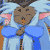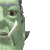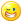## Welcome to the Second Life Forums Archive

These forums are CLOSED. Please visit the new forums HERE

# Discussion: Float2Hex & Float2Sci, safe transport for floats without need of a parser

Strife Onizuka
MoonchildJoin date: 3 Mar 2004
Posts: 5,887
01-25-2006 03:04
This is the support thread for two Scripts i've written, Float2Hex & Float2Sci.

SL support the C99 style for encoding floats as a hex string.
This method for encoding provides many advantages, 1) the data is stored in a memory in such a way to conserve it 2) the function is fast with a speed of 0.11 seconds per iteration. The number doesn't effect the speed.

This function could be better written to conserve memory by adding a decimal point into the string for numbers around zero, this could free up a couple of bytes. It is a low priority. Doing so would come at quite a bit of expense in speed. Also any gaining those couple extra bytes, many more bytes would be wasted in bytecode. There are some small optimizations that can be made. The zero stripper is needed or "p" is ignored.

CODE
`string hexc="0123456789ABCDEF";//fasterstring Float2Hex(float a){// Copyright Strife Onizuka, 2006, LGPL, http://www.gnu.org/copyleft/lesser.html    if(a)    {        float b = llFabs(a);//logs don't work on negatives.        string f = "p";        integer c = llFloor(llLog(b) / 0.69314718055994530941723212145818);//floor(log2(b))        if(c > 127) c = 127;//catch fatal rounding error in exponent.        integer d = (integer)((b / (float)("0x1p"+(string)c)) * 0x1000000);//shift up into integer range        while(!(d & 0xf))        {//strip extra zeros off before converting or they break "p"            d = d >> 4;            c+=4;        }        do            f = llGetSubString(hexc,15&d,15&d) + f;        while(d = d >> 4);        if(a < 0)            return "-0x" + f +(string)(c - 24);        return "0x" + f +(string)(c - 24);    }    return "0";//zero would hang the zero stripper.}`

Float2Sci: /15/28/28006/1.html

Float2Sci is slower then Float2Hex and should only be used where hex-floats are not support or easily readable (hex-floats are not very human readable).

CODE
` string Float2Sci(float input){    if(input == 0.0)//handles negitive zero        return llDeleteSubString((string)input, -5, -1);//trim off the trailing zero's, don't need them.        float frac = llFabs(input);//we put the negitive back at the end.    string mantissa = (string)frac;//this may be a string of about 47 characters long.    integer exponent = -6;//default exponent for optical method    if(frac < 16.0)//optical might fail        if(frac != (float)mantissa)//optical did fail            jump in;    //optical will work, so all we need do is remove the decimal point and jump to optical.    mantissa = llDeleteSubString(mantissa, -7, -7);    jump optical;    @in;    //Ugly Math version; ugly in the sence that it is slow and not as elegant as working with it as a string.    //A) calculate the exponent via approximation of C log2()    //B) use cludge to avert fatal error in approximation of log2 result (only a problem with values >= 0x1.FFFFF8p127)    //       the exponent is sometimes reported as 128, which will bork float math, so we subtract the test for 128.    //       max_float btw is 0x1.FFFFFEp127, so we are only talking a very small number of numbers.    //C) normalize the float with questionable exponent    //D) calculate rounding error left from log2 approimation and add to normalization value.    //       the '|' acts like a '+' in this instance but saves us one byte.    integer position = (24 | (3 <= frac)) - (integer)( //D            frac /= (float)("0x1p"+(string)( //C            exponent = (exponent - (( //B                exponent = llFloor(llLog(frac) / 0.69314718055994530941723212145818) //A            ) == 128))            ))            );        //this pushes the float into the interger buffer exactly.    //since the shift is within integer range, we don't need to make a float.    integer int = (integer)(frac * (1 << position));    integer target = (integer)(frac = 0.0);//since the float is in the integer buffer, we need to clear the float buffer.        //we don't use a traditional while loop, and instead opt for a do-while, because it's faster    //since we may have to do about 128 iteration, this savings is important,    //the exponent needs one final adjustment because of the shift, we do it here to save memory & it's fasfter.        //The two loops try to make exponent == position by shifting and multiplying.    //when they are equal, then this should be true ((int * llPow(10, exponent)) == llFabs(input))    //That is of course assuming that the llPow(10, exponenet) result has enough percision.        //We recycle position for these loops as a temporary buffer. This is so we can save a few operations.    //If we didn't, then we could actualy optimize the variable out of the code; though it would be slower.    if(target > (exponent -= position))    {//apply the rest of the bit shift if |input| < 1        do        {            if(int < 0x19999999)//(0x80000000 / 5)            {//won't overflow, multiply in 5                int = int * 5 + (position = (integer)(frac *= 5.0));                frac -= (float)position;                target = ~-target;            }            else            {//overflow predicted, devide by 2                frac = (frac + (int & 1))/2;                int = int >> 1;                exponent = -~exponent;            }        }while(target ^ exponent);    }    else if(target ^ exponent)//target < exponent    {//apply the rest of the bit shift if |input| > 1        do        {            if(int < 0x40000000) //(0x80000000 / 2)            {//won't overflow, multiply in 2                int = (int << 1) + (position = (integer)(frac *= 2.0));                frac -= (float)position;                exponent = ~-exponent;            }            else            {//overflow predicted, devide by 5                frac = (frac + int%5) / 5.0;                int /= 5;                target = -~target;            }        }while(target ^ exponent);    }    //int is now properly calculated, it holds enough data to accurately describe the input in conjunction with exponent.    //we feed this through optical to clean up the answer.    mantissa = (string)int;    @optical;    //it's not an issue that we may be jumping over the initialization of some of the variables,    //we initialize everything we use here.        //to accurately describe a float you only need 9 decimal places; so we throw the extra's away    if(9 < (target = position = llStringLength(mantissa)))        position = 9;    //chop off the tailing zero's; we don't need them.    do; while(llGetSubString(mantissa, position, position) == "0" && (position = ~-position));//faster then a while loop        //we do a bad thing, we recycle 'target' here, position is one less then target,    //"target + ~position" is the same as "target - (position + 1)" saves 6 bytes.    //this block of code actualy does the cutting.    if(target + ~position) mantissa = llGetSubString(mantissa, 0, position);        //insert the decimal point (not strictly needed). We add the extra zero for asthetics.    //by adding in the decimal point, it simplifies some of the code.    mantissa = llInsertString(mantissa, 1, llGetSubString(".0", 0, !position));    //adjust exponent from having added the decimal place    if((exponent += ~-target))        mantissa += "e" + (string)exponent;    //return with the correct sign.    if(input < 0)        return "-" + mantissa;    return mantissa;}`
_____________________
Truth is a river that is always splitting up into arms that reunite. Islanded between the arms, the inhabitants argue for a lifetime as to which is the main river.
- Cyril Connolly

Without the political will to find common ground, the continual friction of tactic and counter tactic, only creates suspicion and hatred and vengeance, and perpetuates the cycle of violence.
- James Nachtwey
Nada Epoch
The LibrarianJoin date: 4 Nov 2002
Posts: 1,423
Original Thread
01-25-2006 14:24
/15/09/84363/1.html
_____________________
i've got nothing.Strife Onizuka
MoonchildJoin date: 3 Mar 2004
Posts: 5,887
11-02-2006 14:23
I've updated Float2Sci & put the most current version from the wiki of Float2Hex here.

The page for Float2Hex on the wiki is:
http://lslwiki.com/lslwiki/wakka.php?wakka=LibraryFloat2Hex
_____________________
Truth is a river that is always splitting up into arms that reunite. Islanded between the arms, the inhabitants argue for a lifetime as to which is the main river.
- Cyril Connolly

Without the political will to find common ground, the continual friction of tactic and counter tactic, only creates suspicion and hatred and vengeance, and perpetuates the cycle of violence.
- James Nachtwey